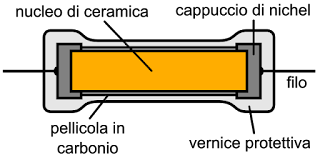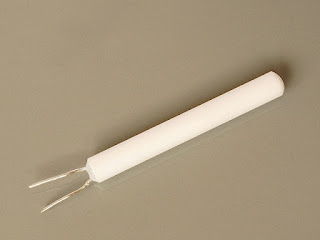## What is a resistor, Types of a resistor, application of resistor?

The resistor is a very important, useful, basic component in electrical and electronics circuits. It is very much known to us.
Resistor: Resistor is a component which resists the flow of current in an electrical circuit.

### Types of Resistor:

#### Resistors are basically two types-

1. Linear Resistor: If the value of resistance of the resistor does not change with the applied voltage, and temperature then the resistor is called a linear resistor.

Linear resistors are two types,
1.1. Fixed resistors (This type resistor has a fixed value that means the value cannot be changed)

Types of Fixed resistors,
1.1.1. Thick Film Resistors (the film or layer of resistance material is thick)
1.1.2. Thin Film Resistors (the film or layer of resistance material is thin)
1.1.3. Carbon Composition ResistorsCarbon Film Resistor Image from Wikimedia

1.1.4. Wire Wound Resistors
1.2. Variable resistors (The value of this type of resistors can be changed)
Types of Variable Resistors,
1.2.1. Potentiometers
1.2.2. Rheostats
1.2.3. TrimmersWire Wound Resistor image from Wikimedia

2. Non- Linear Resistor:  If the value of resistance of the resistor changes with the applied voltage, the temperature then the resistor is called a linear resistor. As the resistance changes, the current flowing through the resistor also changes according to OHM’s Law.

Types of Non- Linear Resistor,
2.1. Thermisters (it's valued changes with temperature)
2.2. Voltage-dependent resistor (VDR)
2.3. Light depended on resistors (LDR) - it's valued changes with light intensity.

### Application of Resistors:

Many uses of resistors are there. The main application of resistors is current control or limiting. It can convert electrical energy to heat energy.

What is resistor, Types of resistor, application of resistorReviewed by manoranjan das on 10/14/2018 Rating: 5